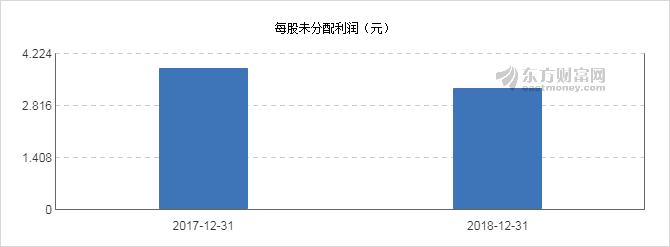@nbsp@格尔软件5月24日公布2018年年度分红派息实施方案：以本公司总股本8540万股为基数，向全体股东每10股转增4.2股、派发现金红利2.8元（含税），合计派发现金红利总额为2391万元。

本次权益分派股权登记日为2019年5月29日，除权除息日为2019年5月30日。格尔软件2018年年报显示，截至2018年12月31日，公司的营业收入3.09亿元，同比增长13.6%，净利润7180万，同比增长2.21%；每股收益0.84元，每股净资产7.28元，净资产收益率11.77%。近年来，公司历次分红派息情况如下表所示：

2018-12 2019-04-20 10转4.20派2.80元 0.79 0.84 3.28 -26.86 05-29 0.43 05-30 -
2017-12 2018-04-28 10转4.00派4.00元 0.95 1.26 3.84 -6.86 06-14 -11.38 06-15 -6.82

近期，英派斯华天科技恒林股份隆基股份华建集团分别公布了分红方案，具体情况如下表：

(%)

(元)

002899 英派斯 10派0.94元 0.66 0.52 1.9 -24.63 04-30 05-29
002185 华天科技 10派0.20元 0.35 0.18 1.03 -21.27 04-30 05-30
603661 恒林股份 10派2.80元 0.8 1.71 7.57 3.15 04-30 -
601012 隆基股份 10派1.00元 0.44 0.93 2.86 -28.24 04-30 -
600629 华建集团 10转2.00派1.80元 1.31 0.61 2.49 2.78 04-30 -

近年来，格尔软件每股未分配利润情况如下图：同期，该公司的每股公积金和股息率情况如下图：公司自上市以来分红派现及募集资金的对比情况如下图：(注：数据来源东方财富Choice数据，截至2019-05-24)

(责任编辑：DF062)

（责任编辑：士剑波）

## 联众世界棋牌 相关新闻

• <<<<<<<<<|
• <<<<<<<<<
• <<<<<<<<<
• <<<<<<<<<
• <<<<<<<<<
• <<<<<<<<<
• <<<<<<<<<
• <<<<<<<<<
• <<<<<<<<<
<<<<<<<<< <<<<<<<<<

• <<<<<<<<<
• <<<<<<<<<
• <<<<<<<<<
• <<<<<<<<<
• <<<<<<<<<
• <<<<<<<<< <<<<<<<<<
• <<<<<<<<< <<<<<<<<<

• <<<<<<<<<
• <<<<<<<<<
• <<<<<<<<<
• <<<<<<<<<
• <<<<<<<<<
• <<<<<<<<<
• <<<<<<<<<

<<<<<<<<<快加入我们吧！

025-58505216

<<<<<<<<<

• 工业品
• 制造业
• 原材料
• 商务服务
<<<<<<<<<
<<<<<<<<<

102172 2

<<<<<<<<<

<<<<<<<<<

<<<<<<<<<2条 >>

<<<<<<<<<
<<<<<<<<<

100531 1

<<<<<<<<<

<<<<<<<<<

TD75型皮带输送机厂家

<<<<<<<<<1条 >>

<<<<<<<<<
<<<<<<<<<

91773 1

<<<<<<<<<

<<<<<<<<<

<<<<<<<<<1条 >>

<<<<<<<<<
<<<<<<<<<

86444 1

<<<<<<<<<

<<<<<<<<<

<<<<<<<<<1条 >>

<<<<<<<<<
<<<<<<<<<

107671 1

<<<<<<<<<

<<<<<<<<<

<<<<<<<<<1条 >>

<<<<<<<<<
<<<<<<<<<

99238 1

<<<<<<<<<

<<<<<<<<<

<<<<<<<<<1条 >>

<<<<<<<<<
<<<<<<<<<

80333 1

<<<<<<<<<

<<<<<<<<<

<<<<<<<<<1条 >>

<<<<<<<<<
<<<<<<<<<

77143 1

<<<<<<<<<

<<<<<<<<<

<<<<<<<<<1条 >>

<<<<<<<<<
<<<<<<<<<

93054 1

<<<<<<<<<

<<<<<<<<<

PSP钢塑复合压力管

<<<<<<<<<1条 >>

<<<<<<<<<
<<<<<<<<<

85418 1

<<<<<<<<<

<<<<<<<<<

<<<<<<<<<1条 >>

<<<<<<<<<
<<<<<<<<<

84335 1

<<<<<<<<<

<<<<<<<<<

<<<<<<<<<1条 >>

<<<<<<<<<
<<<<<<<<<

81261 1

<<<<<<<<<

<<<<<<<<<

<<<<<<<<<1条 >>

<<<<<<<<<
<<<<<<<<<

42136 1

<<<<<<<<<

<<<<<<<<<

<<<<<<<<<1条 >>

<<<<<<<<<
<<<<<<<<<

39566 1

<<<<<<<<<

<<<<<<<<<

<<<<<<<<<1条 >>

<<<<<<<<<
<<<<<<<<<

36330 1

<<<<<<<<<

<<<<<<<<<

<<<<<<<<<1条 >>

<<<<<<<<<
<<<<<<<<<

30066 1

<<<<<<<<<

<<<<<<<<<

<<<<<<<<<1条 >>

<<<<<<<<<
<<<<<<<<<
F1  机械设备
<<<<<<<<<
<<<<<<<<<

7335 0

<<<<<<<<<
<<<<<<<<<

107671 1

<<<<<<<<<

<<<<<<<<<

<<<<<<<<<1条 >>

<<<<<<<<<
<<<<<<<<<

7115 0

<<<<<<<<<
<<<<<<<<<

561 1

<<<<<<<<<

<<<<<<<<<

<<<<<<<<<1条 >>

<<<<<<<<<
<<<<<<<<<

593 1

<<<<<<<<<

<<<<<<<<<

<<<<<<<<<1条 >>

• <<<<<<<<<
• <<<<<<<<<
• <<<<<<<<<
• <<<<<<<<<
• <<<<<<<<<
• <<<<<<<<<
• <<<<<<<<<
F2  五金工具
<<<<<<<<<
<<<<<<<<<

693 1

<<<<<<<<<

<<<<<<<<<

<<<<<<<<<1条 >>

<<<<<<<<<
<<<<<<<<<

11338 1

<<<<<<<<<

<<<<<<<<<

<<<<<<<<<1条 >>

<<<<<<<<<
<<<<<<<<<

9827 1

<<<<<<<<<

<<<<<<<<<

<<<<<<<<<1条 >>

<<<<<<<<<
<<<<<<<<<

495 1

<<<<<<<<<

<<<<<<<<<

<<<<<<<<<1条 >>

<<<<<<<<<
<<<<<<<<<

475 1

<<<<<<<<<

<<<<<<<<<

<<<<<<<<<1条 >>

• <<<<<<<<<
• <<<<<<<<<
• <<<<<<<<<
• <<<<<<<<<
• <<<<<<<<<
• <<<<<<<<<
• <<<<<<<<<
F3  化工设备
<<<<<<<<<
<<<<<<<<<

669 0

<<<<<<<<<
<<<<<<<<<

330 1

<<<<<<<<<

<<<<<<<<<

<<<<<<<<<1条 >>

<<<<<<<<<
<<<<<<<<<

5986 1

<<<<<<<<<

<<<<<<<<<

<<<<<<<<<1条 >>

<<<<<<<<<
<<<<<<<<<

8491 1

<<<<<<<<<

<<<<<<<<<

<<<<<<<<<1条 >>

<<<<<<<<<
<<<<<<<<<

496 1

<<<<<<<<<

<<<<<<<<<

<<<<<<<<<1条 >>

• <<<<<<<<<
• <<<<<<<<<
• <<<<<<<<<
• <<<<<<<<<
• <<<<<<<<<
• <<<<<<<<<
• <<<<<<<<<
F4  仪器仪表
<<<<<<<<<
<<<<<<<<<

433 1

<<<<<<<<<

<<<<<<<<<

<<<<<<<<<1条 >>

<<<<<<<<<
<<<<<<<<<

5483 1

<<<<<<<<<

<<<<<<<<<

<<<<<<<<<1条 >>

<<<<<<<<<
<<<<<<<<<

5630 1

<<<<<<<<<

<<<<<<<<<

mai@东莞市科宝试验设备有限公司

<<<<<<<<<1条 >>

<<<<<<<<<
<<<<<<<<<

376 1

<<<<<<<<<

<<<<<<<<<

<<<<<<<<<1条 >>

<<<<<<<<<
<<<<<<<<<

6262 1

<<<<<<<<<

<<<<<<<<<

<<<<<<<<<1条 >>

• <<<<<<<<<
• <<<<<<<<<
• <<<<<<<<<
• <<<<<<<<<
• <<<<<<<<<
• <<<<<<<<<
• <<<<<<<<<
F5  数码家电
<<<<<<<<<
<<<<<<<<<

8288 1

<<<<<<<<<

<<<<<<<<<

<<<<<<<<<1条 >>

<<<<<<<<<
<<<<<<<<<

67717 1

<<<<<<<<<

<<<<<<<<<

<<<<<<<<<1条 >>

<<<<<<<<<
<<<<<<<<<

50287 1

<<<<<<<<<

<<<<<<<<<

<<<<<<<<<1条 >>

<<<<<<<<<
<<<<<<<<<

50119 1

<<<<<<<<<

<<<<<<<<<

<<<<<<<<<1条 >>

<<<<<<<<<
<<<<<<<<<

5482 0

• <<<<<<<<<
• <<<<<<<<<
• <<<<<<<<<
• <<<<<<<<<
• <<<<<<<<<
• <<<<<<<<<
• <<<<<<<<<
F6  服装饰品
<<<<<<<<<
<<<<<<<<<

5732 1

<<<<<<<<<

<<<<<<<<<

<<<<<<<<<1条 >>

<<<<<<<<<
<<<<<<<<<

3213 1

<<<<<<<<<

<<<<<<<<<

<<<<<<<<<1条 >>

<<<<<<<<<
<<<<<<<<<

5732 1

<<<<<<<<<

<<<<<<<<<

<<<<<<<<<1条 >>

<<<<<<<<<
<<<<<<<<<

4206 1

<<<<<<<<<

<<<<<<<<<

<<<<<<<<<1条 >>

<<<<<<<<<
<<<<<<<<<

2983 2

<<<<<<<<<

<<<<<<<<<

<<<<<<<<<2条 >>

• <<<<<<<<<
• <<<<<<<<<
• <<<<<<<<<
• <<<<<<<<<
• <<<<<<<<<
• <<<<<<<<<
• <<<<<<<<<
F7  电子电工
<<<<<<<<<
<<<<<<<<<

4200 1

<<<<<<<<<

<<<<<<<<<

<<<<<<<<<1条 >>

<<<<<<<<<
<<<<<<<<<

2211 1

<<<<<<<<<

<<<<<<<<<

<<<<<<<<<1条 >>

<<<<<<<<<
<<<<<<<<<

6581 1

<<<<<<<<<

<<<<<<<<<

<<<<<<<<<1条 >>

<<<<<<<<<
<<<<<<<<<

10526 1

<<<<<<<<<

<<<<<<<<<

<<<<<<<<<1条 >>

<<<<<<<<<
<<<<<<<<<

2167 1

<<<<<<<<<

<<<<<<<<<

<<<<<<<<<1条 >>

• <<<<<<<<<
• <<<<<<<<<
• <<<<<<<<<
• <<<<<<<<<
• <<<<<<<<<
• <<<<<<<<<
• <<<<<<<<<
F8  行业材料
<<<<<<<<<
<<<<<<<<<

623 0

<<<<<<<<<
<<<<<<<<<

632 1

<<<<<<<<<

<<<<<<<<<

<<<<<<<<<1条 >>

<<<<<<<<<
<<<<<<<<<

7245 1

<<<<<<<<<

<<<<<<<<<

<<<<<<<<<1条 >>

<<<<<<<<<
<<<<<<<<<

1098 1

<<<<<<<<<

<<<<<<<<<

<<<<<<<<<1条 >>

<<<<<<<<<
<<<<<<<<<

6607 1

<<<<<<<<<

<<<<<<<<<

<<<<<<<<<1条 >>

• <<<<<<<<<
• <<<<<<<<<
• <<<<<<<<<
• <<<<<<<<<
• <<<<<<<<<
• <<<<<<<<<
• <<<<<<<<<
F9  工艺礼品
<<<<<<<<<
<<<<<<<<<

608 1

<<<<<<<<<

<<<<<<<<<

<<<<<<<<<1条 >>

<<<<<<<<<
<<<<<<<<<

591 1

<<<<<<<<<

<<<<<<<<<

<<<<<<<<<1条 >>

<<<<<<<<<
<<<<<<<<<

3771 1

<<<<<<<<<

<<<<<<<<<

<<<<<<<<<1条 >>

<<<<<<<<<
<<<<<<<<<

783 1

<<<<<<<<<

<<<<<<<<<

<<<<<<<<<1条 >>

<<<<<<<<<
<<<<<<<<<

22856 1

<<<<<<<<<

<<<<<<<<<

f014@星世乐游乐设备

<<<<<<<<<1条 >>

• <<<<<<<<<
• <<<<<<<<<
• <<<<<<<<<
• <<<<<<<<<
• <<<<<<<<<
• <<<<<<<<<
• <<<<<<<<<
F10  商务服务
<<<<<<<<<
<<<<<<<<<

475 1

<<<<<<<<<

<<<<<<<<<

<<<<<<<<<1条 >>

<<<<<<<<<
<<<<<<<<<

843 1

<<<<<<<<<

<<<<<<<<<

<<<<<<<<<1条 >>

<<<<<<<<<
<<<<<<<<<

11049 1

<<<<<<<<<

<<<<<<<<<

<<<<<<<<<1条 >>

<<<<<<<<<
<<<<<<<<<

8671 1

<<<<<<<<<

<<<<<<<<<

<<<<<<<<<1条 >>

<<<<<<<<<
<<<<<<<<<

1158 1

<<<<<<<<<

<<<<<<<<<

<<<<<<<<<1条 >>

• <<<<<<<<<
• <<<<<<<<<
• <<<<<<<<<
• <<<<<<<<<
• <<<<<<<<<
• <<<<<<<<<
• <<<<<<<<<
<<<<<<<<<
<<<<<<<<<
<<<<<<<<<

<<<<<<<<<
• <<<<<<<<<
• <<<<<<<<<
• <<<<<<<<<
• <<<<<<<<<
• <<<<<<<<<
• <<<<<<<<<
• <<<<<<<<<

<<<<<<<<<

<<<<<<<<<

• <<<<<<<<<
• <<<<<<<<<
• <<<<<<<<<
• <<<<<<<<<

• <<<<<<<<<
• <<<<<<<<<
• <<<<<<<<<
• <<<<<<<<<
• <<<<<<<<<
• <<<<<<<<<
• <<<<<<<<<
• 机械五金
<<<<<<<<<
<<<<<<<<<
<<<<<<<<<
<<<<<<<<<
<<<<<<<<<
<<<<<<<<<
<<<<<<<<<
<<<<<<<<<
<<<<<<<<<
<<<<<<<<<
<<<<<<<<<
<<<<<<<<<
• 仪器仪表
<<<<<<<<<
<<<<<<<<<
<<<<<<<<<
<<<<<<<<<
<<<<<<<<<
<<<<<<<<<
<<<<<<<<<
<<<<<<<<<
<<<<<<<<<
<<<<<<<<<
<<<<<<<<<
<<<<<<<<<
• 化工设备
<<<<<<<<<
<<<<<<<<<
<<<<<<<<<
<<<<<<<<<
<<<<<<<<<
<<<<<<<<<
<<<<<<<<<
<<<<<<<<<
<<<<<<<<<
<<<<<<<<<
<<<<<<<<<
<<<<<<<<<
• 原材料
<<<<<<<<<
<<<<<<<<<
<<<<<<<<<
<<<<<<<<<
<<<<<<<<<
<<<<<<<<<
<<<<<<<<<
<<<<<<<<<
<<<<<<<<<
<<<<<<<<<
<<<<<<<<<
<<<<<<<<<
• 商务服务
<<<<<<<<<
<<<<<<<<<
<<<<<<<<<
<<<<<<<<<
<<<<<<<<<
<<<<<<<<<
<<<<<<<<<
<<<<<<<<<
<<<<<<<<<
<<<<<<<<<
<<<<<<<<<
<<<<<<<<<
• 消费品
<<<<<<<<<
<<<<<<<<<
<<<<<<<<<
<<<<<<<<<
<<<<<<<<<
<<<<<<<<<
<<<<<<<<<
<<<<<<<<<
<<<<<<<<<
<<<<<<<<<
<<<<<<<<<
<<<<<<<<<
<<<<<<<<<
01社交营销

02三端同步

WEB端、PC端、手机端

03搜索引擎优化

• 关于我们

<<<<<<<<<

<<<<<<<<<

<<<<<<<<<

<<<<<<<<<

<<<<<<<<<

<<<<<<<<<

• 产品中心

<<<<<<<<<

<<<<<<<<<

<<<<<<<<<

• 渠道中心

<<<<<<<<<

<<<<<<<<<

<<<<<<<<<

<<<<<<<<<

<<<<<<<<<

• 帮助中心

<<<<<<<<<

<<<<<<<<<

<<<<<<<<<

<<<<<<<<<

<<<<<<<<<

• 常见问题

<<<<<<<<<

<<<<<<<<<

<<<<<<<<<

<<<<<<<<<

<<<<<<<<<

<<<<<<<<<

• 烽火台服务咨询

客服： <<<<<<<<<

联系电话：025-58505216

• 扫一扫下载烽火台app
玩转商业分享

<<<<<<<<< <<<<<<<<< <